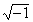Celebrity Numbers

 The numbersare celebrities among numbers. They are "more famous" and very useful and important. The numbersare probably familiar to you. The numbers e and i may not be familiar.1, One One is the oldest number. As the smallest counting number it is the number humans created first. To the Greeks, one was unity for it is a factor of every number. It the only natural number that is neither prime or composite, because it is unity. It is the multiplicative identity because the product of one and a number is that number. One is its own reciprocal. Any number to the power one is that number. So, it is true that.0, Zero Zero is the only whole number which is not a counting number. The whole numbers differ from counting numbers only because of zero. It is the only number that is neither positive nor negative. It is its own opposite. It is the only number without a reciprocal, since division by zero is undefined [see UNDEFINED]. Zero is the additive identity since its sum with a number is the number. Playfully, creatively, using the number 1 and an operations, one might make the statement: 1 - 1 = 0, thus relating it to another celebrity number.-1, Negative One The opposite of one, the additive inverse of one, is negative one. Its product with a number is the number's opposite. Negative one is its own reciprocal. Playfully, creatively, one might make the statement, 0 - 1 = -1 or -1 + 1 = 0, thus relating -1 to two other celebrity numbers and making basic but important statements as well.i, The "Imaginary" Unit The number i, named for the first letter of the word imaginary, is the square root of negative one,. It solves the equationwith solutions of i and -i. Each complex number is the sum of an imaginary number and a real number, a + bi, where a and b are real. With pure imaginary numbers, a is zero. With pure real numbers, b is zero. Multiples of i include every pure imaginary number. Powers of i include only: i, -1, -i, and 1.The letter pi is the first letter of the Greek word for periphery or circumference. It is the symbol for the ratio of a circle's circumference to its diameter. Radian angle measures highlight the importance of this number. The sine of pi is zero:. The cosine of pi is one:. Because of the periodicity of the circle, the sine, and the cosine, where n is a natural number,.e, The Base of the Natural Log The number e is about equal to 2.71828. Leonard Euler conventionalized the symbol for e as he did for the numbersand i. But stating who chose the symbol does not explain the reason for naming this number in such a manner.One explanation involves an area. It's an important area because it conveniently relates logarithmic and exponential functions to rational and polynomial functions. There are many log and exponential functions because there are many choices for bases. Ten, the base of our number system, is much too large. Nine, eight, and six are all too large to make the slope of the reciprocal function equal to a log function. But, there is a number to serve as a base which makes the slope of the reciprocal function equal to a log function. The ideal base is the number that makes the area described below equal to 1. Consider the graph of the horizontal line y = 0 as a bottom boundary line. Use the vertical line x = 1 as a left boundary line, and the reciprocal function as a top boundary line. A four-sided shape would be created if a vertical line were drawn on the right. The vertical line which creates a shape having an area of one is the vertical line x = e. With this number e as the base, the slope of the reciprocal function is the log function the because e provides the exact curvature to make that happen. This is really important and useful. The number e is also useful in writing complex numbers. Using Abraham DeMoivre's Formula for the computation of complex powers,, Euler profoundly stated,. With his profound statement four of our celebrity numbers are related. The End Now, we playfully end this dictionary relating all these celebrity numbers..

This is a page from the dictionary MATH SPOKEN HERE!, published in 1995 by MATHEMATICAL CONCEPTS, inc., ISBN: 0-9623593-5-1.   You are hereby granted permission to make ONE printed copy of this page and its picture(s) for your PERSONAL and not-for-profit use.